# 12 Results

View
Selected filters:
• CCSS.Math.Content.8.G.B.6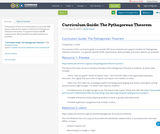Conditional Remix & Share Permitted
CC BY-NC
Rating

The purpose of this curriculum guide is to provide OER lesson material and support activities for Pythagorean Theorem instruction. It is geared towards GED® requirements. Both printable and online options are provided.

Subject:
Mathematics
Material Type:
Activity/Lab
Assessment
Lesson
Teaching/Learning Strategy
Author:
Shana Friend
05/30/2018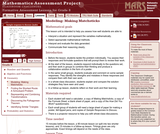Only Sharing Permitted
CC BY-NC-ND
Rating

This lesson unit is intended to help you assess how well students are able to: interpret a situation and represent the variables mathematically; select appropriate mathematical methods; interpret and evaluate the data generated; and communicate their reasoning clearly.

Subject:
Mathematics
Material Type:
Assessment
Lesson Plan
Provider:
Shell Center for Mathematical Education
U.C. Berkeley
Provider Set:
Mathematics Assessment Project (MAP)
04/26/2013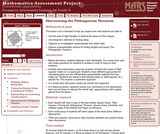Only Sharing Permitted
CC BY-NC-ND
Rating

This lesson unit is intended to help teachers assess how well students are able to: use the area of right triangles to deduce the areas of other shapes; use dissection methods for finding areas; organize an investigation systematically and collect data; deduce a generalizable method for finding lengths and areas (The Pythagorean Theorem.)

Subject:
Geometry
Material Type:
Assessment
Lesson Plan
Provider:
Shell Center for Mathematical Education
U.C. Berkeley
Provider Set:
Mathematics Assessment Project (MAP)
04/26/2013Only Sharing Permitted
CC BY-NC-ND
Rating

This lesson unit is intended to help you assess how students reason about geometry and, in particular, how well they are able to: use facts about the angle sum and exterior angles of triangles to calculate missing angles; apply angle theorems to parallel lines cut by a transversal; interpret geometrical diagrams using mathematical properties to identify similarity of triangles.

Subject:
Geometry
Material Type:
Assessment
Lesson Plan
Provider:
Shell Center for Mathematical Education
U.C. Berkeley
Provider Set:
Mathematics Assessment Project (MAP)
04/26/2013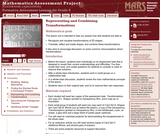Only Sharing Permitted
CC BY-NC-ND
Rating

This lesson unit is intended to help teachers assess how well students are able to: recognize and visualize transformations of 2D shapes; and translate, reflect and rotate shapes, and combine these transformations. It also aims to encourage discussion on some common misconceptions about transformations.

Subject:
Geometry
Material Type:
Assessment
Lesson Plan
Provider:
Shell Center for Mathematical Education
U.C. Berkeley
Provider Set:
Mathematics Assessment Project (MAP)
04/26/2013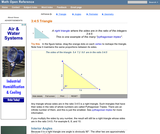Rating

An interactive applet and associated web page that demonstrate the properties of a 3:4:5 triangle - one of the Pythagorean triples. The applet shows a right triangle that can be resized by dragging any vertex. As it is dragged, the remaining vertices change so that the triangle's side remain in the ration 3:4:5. The text on the page has an example of how the triangle can be used to measure a right angle on even large objects. Applet can be enlarged to full screen size for use with a classroom projector. This resource is a component of the Math Open Reference Interactive Geometry textbook project at http://www.mathopenref.com.

Subject:
Geometry
Material Type:
Simulation
Provider:
Math Open Reference
Author:
John Page
02/16/2011
Remix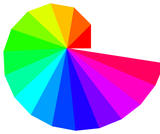Unrestricted Use
CC BY
Rating

This is a cross curricular art project for 8th grade math students. Students are first introduced to what the Wheel of Theodorus is, ponder where they see it in the world around them and then instructed on how to create their own. When they have finished constructing their Wheel of Theodorus they are asked to creatively and colorfully turn it "into" something. Examples are given. After they Wheel of Theodorus is complete, students are then asked to measure all the sides lengths of the triangles in the wheel. They should quickly see that they can use the Pythagorean Theorem to do this and that it follows a predictable pattern. No ruler will be required for this part of the project!

Subject:
Mathematics
Geometry
Material Type:
Activity/Lab
Homework/Assignment
Author:
Jennifer Chandler
Sara Scholes
03/26/2020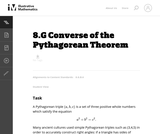Unrestricted Use
CC BY
Rating

This task is for instruction purposes. Part (b) is subtle and the solution presented here uses a "dynamic" view of triangles with two side lengths fixed. This helps pave the way toward what students will see later in trigonometry but some guidance will likely be needed in order to get students started on this path.

Subject:
Mathematics
Geometry
Material Type:
Activity/Lab
Provider:
Illustrative Mathematics
Provider Set:
Illustrative Mathematics
Author:
Illustrative Mathematics
08/17/2012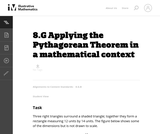Unrestricted Use
CC BY
Rating

This task requires students to apply the Pythagorean Theorem.

Subject:
Mathematics
Geometry
Material Type:
Activity/Lab
Provider:
Illustrative Mathematics
Provider Set:
Illustrative Mathematics
Author:
Illustrative Mathematics
05/01/2012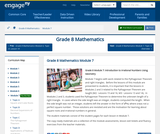Conditional Remix & Share Permitted
CC BY-NC-SA
Rating

Module 7 begins with work related to the Pythagorean Theorem and right triangles.  Before the lessons of this module are presented to students, it is important that the lessons in Modules 2 and 3 related to the Pythagorean Theorem are taught (M2:  Lessons 15 and 16, M3:  Lessons 13 and 14).  In Modules 2 and 3, students used the Pythagorean Theorem to determine the unknown length of a right triangle.  In cases where the side length was an integer, students computed the length.  When the side length was not an integer, students left the answer in the form of x2=c, where c was not a perfect square number.  Those solutions are revisited and are the motivation for learning about square roots and irrational numbers in general.

Subject:
Geometry
Material Type:
Module
Provider:
New York State Education Department
Provider Set:
EngageNY
02/02/2014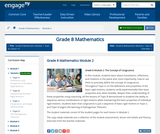Conditional Remix & Share Permitted
CC BY-NC-SA
Rating

In this module, students learn about translations, reflections, and rotations in the plane and, more importantly, how to use them to precisely define the concept of congruence. Throughout Topic A, on the definitions and properties of the basic rigid motions, students verify experimentally their basic properties and, when feasible, deepen their understanding of these properties using reasoning. All the lessons of Topic B demonstrate to students the ability to sequence various combinations of rigid motions while maintaining the basic properties of individual rigid motions. Students learn that congruence is just a sequence of basic rigid motions in Topic C, and Topic D begins the learning of Pythagorean Theorem.

Subject:
Geometry
Material Type:
Module
Provider:
New York State Education Department
Provider Set:
EngageNY
09/21/2013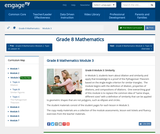Conditional Remix & Share Permitted
CC BY-NC-SA
Rating

In Module 3, students learn about dilation and similarity and apply that knowledge to a proof of the Pythagorean Theorem based on the Angle-Angle criterion for similar triangles.  The module begins with the definition of dilation, properties of dilations, and compositions of dilations.  One overarching goal of this module is to replace the common idea of same shape, different sizes with a definition of similarity that can be applied to geometric shapes that are not polygons, such as ellipses and circles.

Subject:
Geometry
Material Type:
Module
Provider:
New York State Education Department
Provider Set:
EngageNY